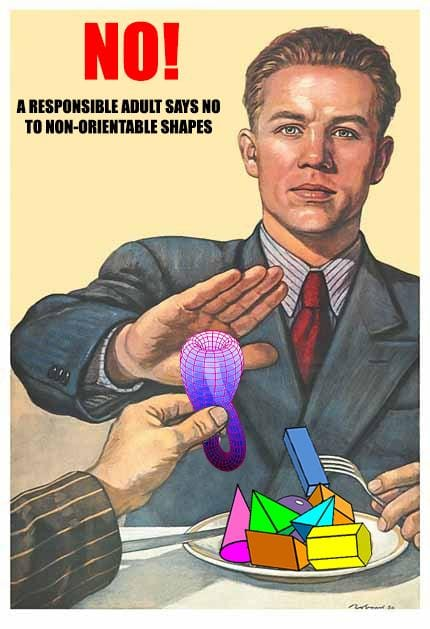David Tong: Vector Calculus

David Tong: Lectures on Vector Calculus

These lectures are aimed at first year undergraduates. They describe the basics of div, grad and curl and various integral theorems. The lecture notes are around 120 pages. Please do email me if you find any typos or mistakes.

PDF

Content

• 1. Curves:   PDF
Tangent vectors and arc lengths, curvature and torsion; Line integrals. Conservative fields and the gradient.
• 2. Surfaces (and Volumes):   PDF
Area integrals and volume integrals, Jacobians, spherical and cylindrical polar coordinates. Flux. The Gauss-Bonnet theorem.
• 3. Grad, Div and Curl:   PDF
The gradient, div, curl; conservative, irrotational and solenoidal fields; the Laplacian. Orthogonal curvilinear coordinates, spherical polar coordinats, cylindrical polar coordinates.
• 4. The Integral Theorems:   PDF
The divergence theorem, conservation laws. Green's theorem in the plane. Stokes' theorem.
• 5. Some Vector Calculus Equations:   PDF
Gravity and electrostatics, Gauss' law and potentials. The Poisson equation and the Laplace equation. Special solutions and the Green's function.
• 6. Tensors:   PDF
Transformation law, maps, and invariant tensors. Tensor fields. A unification of integral theorems.

Problem Sheets

• Problem Sheet 1:   PDF    Grad, div and curl. Line and area integrals.

• Problem Sheet 2:   PDF    Volume integals and integral theorems.

• Problem Sheet 3:   PDF    Changing coordinates. Solving vector calculus equations.

• Problem Sheet 4:   PDF    Feeling tenser.Other Lecture Notes on the Web

• Vector Calculus   previous lecture notes by Ben Allanach and Jonathan Evans

• Vector Calculus   yet earlier lecture notes by Stephen Cowley. Be prepared to draw your own figures!

• Vector Calculus   by Matthias Dorrzapf.

Some History

"I shall conclude by proposing for the consideration of mathematicians certain phrases... I should be greatly obliged to anyone who can give me suggestions on this subject, as I feel that the onomastic power is very faint in me."

• Lecture notes copyright © 2021 David Tong unless otherwise credited. Permission is granted to copy and distribute freely, so long as proper attribution is given, no alterations are made, and no monetary profit is gained.